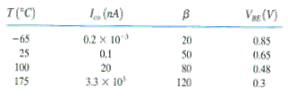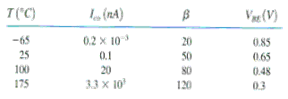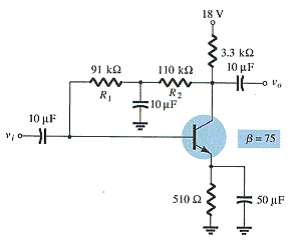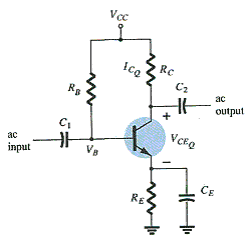# Practice Quiz in DC Biasing – BJT Part 2

(Last Updated On: February 3, 2020)

This is the Online Practice Quiz in DC Biasing – BJTs Part 2 from the book, Electronic Devices and Circuit Theory 10th Edition by Robert L. Boylestad. If you are looking for a reviewer in Electronics Engineering this will definitely help. I can assure you that this will be a great help in reviewing the book in preparation for your Board Exam. Make sure to familiarize each and every questions to increase the chance of passing the ECE Board Exam.

### Continue Part II of the Online Practice Quiz

#### Quiz in DC Biasing – BJTs

Question 11. For an "on" transistor, the voltage VBE should be in the neighborhood of 0.7 V.

A. True

B. False

Question 12. Use this table to determine the change in IC from 25ºC to 175ºC for RB / RE = 250 due to the S(ICO) stability factor. Assume an emitter-bias configuration.A. 140.34 nA

B. 140.34 µA

C. 42.53 nA

D. 0.14034 nA

Question 13. At what region of operation is the base-emitter junction forward biased and the base-collector junction reverse biased?

A. Saturation

B. Linear or active

C. Cutoff

D. None of these

Question 14. Determine the change in IC from 25ºC to 175ºC for the transistor defined in this table for fixed-bias with RB = 240 kΩ and β = 100 due to the S(VBE) stability factor.A. 145.8 µA

B. 145.8 nA

C. –145.8 µA

D. –145.8 nA

Question 15. It is desirable to design a bias circuit that is independent of the transistor beta.

A. True

B. False

Question 16. In a fixed-bias circuit, which one of the stability factors overrides the other factors?

A. S(ICO)

B. S(VBE)

C. S(β)

D. Undefined

Question 17. The saturation region is defined by VCE _____ VCEsat.

A. >

B. <

C. ≤

D. ≥

Question 18. Calculate the value of VCEQ.A. 8.78 V

B. 0 V

C. 7.86 V

D. 18 V

Question 19. For the BJT to operate in the saturation region, the base-emitter junction must be _____-biased and the base-collector junction must be _____-biased.

A. forward, forward

B. forward, reverse

C. reverse, reverse

D. reverse, forward

Question 20. In the case of this circuit, you must assume that VE = 0.1·VCC in order to calculate RC and RE.A. True

B. False

### More Practice Quiz in DC Biasing - BJTs

Practice Quiz Part 1

Practice Quiz Part 2

Practice Quiz Part 3

Practice Quiz Part 4

Practice Quiz Part 5

Practice Quiz Part 6

### See: Complete List of Practice Quizzes

Note: After taking this particular quiz, you can proceed to check all the topics.